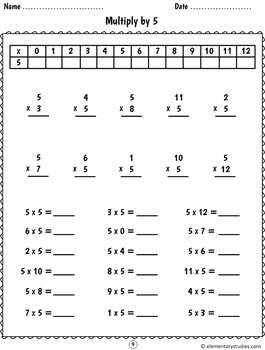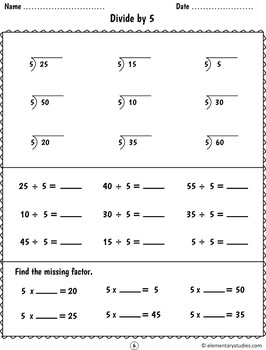# Multiplication and Division Facts | Distance LearningSubject
Resource Type
File Type
Zip (27 MB|98 pages)
\$8.50
List Price:
\$11.00
You Save:
\$2.50
\$8.50
List Price:
\$11.00
You Save:
\$2.50
Product Description
This bundle includes my Multiplication Facts packet and Division Facts packet together in a single zip file.

Contents include:

Multiplication Facts:
Multiplication Facts
---------------------------------------------------------------------------------
Multiplication Facts booklet includes a total of 30 worksheets, 143 Flash Cards, 6 Vocabulary Word Cards and a Multiplication Chart.

* Multiplication Facts Worksheets to practice multiplication skills:
- Make multiplication sentence for addition sentence
- Multiplication equation for Arrays
- Draw array for multiplication equations
- Worksheets for Multiply by 2 till Multiply 12
- Mixed multiplication worksheets
- Story problems to understand the multiplication concept and form a multiplication equation. (An introduction to story problems)
* Multiplication Facts Word Cards: These can be used to make students understand the different concepts of multiplication like Factors, Times, Product and Arrays.
* Flash cards: can be used to help memorize the multiplication facts.
* 12x12 Multiplication chart

Division Facts:
Division Facts
---------------------------------------------------------------------------------
Division facts booklet includes a total of 26 worksheets and a Division fact Vocabulary Chart. Included division sheets for dividing by 1-12

Following division facts worksheets are included to practice division skills:

- Division worksheets for Divide by 2 till Divide by 12 (12 worksheets)
- Mixed Division worksheets (Division 1-12)
- Division Vocabulary Chart
- Division equation for Arrays
- Exploring division story problems to understand the division concept
- Division as repeated subtraction
- Write Division facts from multiplication facts
- Write Division facts and Multiplication facts from fact triangle
Total Pages
98 pages